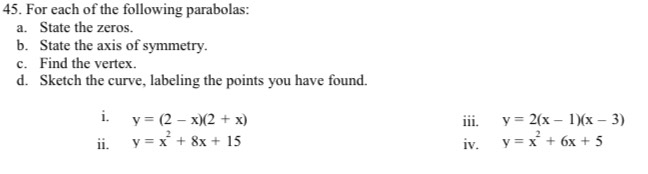### ¿Todavía tienes preguntas de matemáticas?

Pregunte a nuestros tutores expertos
Algebra
Pregunta45. For each of the following parabolas: a. State the zeros. b. State the axis of symmetry. c. Find the vertex. d. Sketch the curve, labeling the points you have found.

i. $$y = ( 2 - x ) ( 2 + x )$$

ii. $$y = x ^ { 2 } + 8 x + 15$$

iii. $$y = 2 ( x - 1 ) ( x - 3 )$$

iv. $$y = x ^ { 2 } + 6 x + 5$$